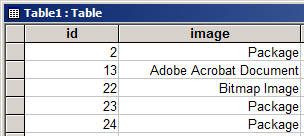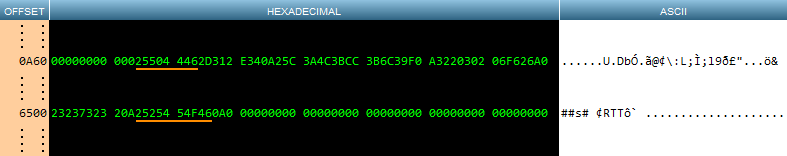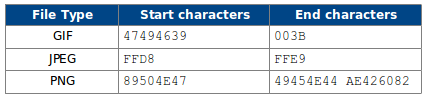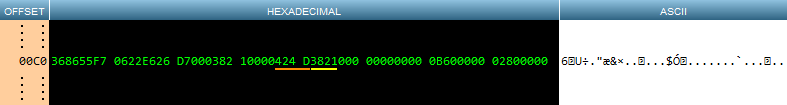# Extract Objects from an Access Database with PHP, Part 2

In the first part of this series we learned how to extract packaged objects from a legacy Access database. In this second part we’ll learn how to extract Acrobat PDF documents and take a brief look at a selection of image formats.

The only similarity that PDF, GIF, PNG, etc., have when stored in an Access database is that they are all wrapped in an OLE container consisting of a variable length header and trailer. As we shall see, the trailer can be ignored as it was with the package discussed in Part 1. The header is more useful, but doesn’t contain all the information we need.

In the test database used for this article, there’s a PDF stored in record number 13:The identification of an Adobe Acrobat Document (PDF) in an Access database OLE object field is achieved using the same method we learned in Part 1 – inspect the first fifty or so bytes looking for a recognisable sequence of characters.

Applying the same method of extracting a chunk of data from the OLE field, then converting it from hexadecimal to decimal, and displaying it in a hex viewer, makes it clear that although we definitely have a PDF stored in the field, we have no other information such as the original name of the PDF or its original file size:Fortunately, there’s another technique we can use to extract the PDF. Every PDF always starts with the character sequence %PDF and ends with the sequence %%END. This can be verified easily by loading a small PDF in a plain text editor. Alternatively, load one into a hex viewer:Here I’ve shown only the first and last few bytes, as these are where the PDF delimiters are. The next step is to apply this technique to the contents of the OLE field and find the location of the start and end and of the embedded PDF:Note that it’s the hexadecimal character sequences that we need to search for; that is, when using PHP’s `strpos()` function to find the start of the embedded PDF, look for 25504446. When looking for the end, 2525454F46 is the relevant character sequence.

So what do we have now? We’ve identified the OLE object as type Acrobat Document, and we have the start and end locations of the embedded file. We thus have all we need to extract the original file from the OLE field using PHP’s `substr()` function.

## Other Object Types

Before discussing popular images types, it’s worth taking a moment to improve the `switch` statement presented in Part 1. For the catch-all default condition we simply displayed a message. It’d be much more useful if any unknown OLE types were extracted and saved to disk for later analysis. The new function `extractUnknown()` takes the entire contents of the OLE field, converts it from hexadecimal to decimal, then saves it to disk using the record ID as its file name. This allows us to view any unknown OLE type in a hex viewer later to ascertain the type of the embedded object. We’ll need this in the next section to identify which records have embedded images.

``````<?php
function extractUnknown(\$id, \$data) {
// convert entire object to decimal and save to disk
file_put_contents(\$id . ".txt", hex2bin(\$data));
}``````

## Popular Image Types

It’s not possible to say with any certainty how image types will be identified in an OLE header in any given Access database. It may be that images will be identified as “Paint Shop Pro 6”, or perhaps they’ll be associated with some other image editing software. It depends entirely on what software was known to the system that was used to store the images and on any file associations that were configured.

In order to know what these unknown types are, we can make a list by running every record with an unknown OLE type through the `extractUnknown()` function. This will be different across the plethora of legacy Access database that exist today.

The image formats we’ll consider here are BMP, GIF, JPEG, and PNG.

### GIF, JPEG, PNG

“Good news, everyone!” These three image types can be processed in exactly the same way. What’s more, it’s exactly the same method used earlier to extract an embedded PDF. First we need to find the start position of the embedded image, then the end position, then extract inclusively everything between these two points. The difference is in how we identify the embedded object. The following table summarises these crucial details:### BMP

Identifying the delimiters of an embedded BMP is similar, but requires a little more work. Finding the start position is as easy as it is with the file types discussed above, but finding the end position requires a bit of math. Let’s see the two crucial elements in a hex viewer:The first two bytes (BM in ASCII), underlined in orange, are the start location of the embedded BMP. The following two bytes, underlined in yellow, are the original size of the BMP stored in little-endian format. The size needs to be converted to big-endian format, then multiplied by two because the object is stored in hexadecimal format.

Given that we now have the start position and the size of the embedded object, we can used the same method to extract a BMP as we used in Part 1 to extract an object from a package.

## Putting It All Together

What follows is the PHP script from Part 1 updated to include the new functionality described above. The basic structure is identical to its previous version, and the additional conditions in the switch() statement shows how easy it is to extend the core logic of the script to accommodate other OLE types.

``````<?php \$offset = array(     "Packager Shell Object" =--> 168,
"Package" => 140
);

if (!function_exists("hex2bin")) {
function hex2bin(\$hexStr) {
\$hexStrLen = strlen(\$hexStr);
\$binStr = "";
\$i = 0;
while (\$i < \$hexStrLen) {             \$a = substr(\$hexStr, \$i, 2);             \$c = pack("H*", \$a);             \$binStr .= \$c;             \$i += 2;        }        return \$binStr;     } } \$dbName = "db1.mdb"; \$db = new PDO("odbc:DRIVER={Microsoft Access Driver (*.mdb)}; DBQ=\$dbName; Uid=; Pwd=;"); \$sql = "SELECT * FROM Table1"; foreach (\$db->query(\$sql) as \$row) {
\$objName = "";

switch (getOLEType(\$row["image"])) {
case "Packager Shell Object":
list(\$objName, \$objData) = extractPackage(\$row["image"], \$offset["Packager Shell Object"]);
break;
case "Package":
list(\$objName, \$objData) = extractPackage(\$row["image"], \$offset["Package"]);
break;
case "Acrobat Document":
list(\$objName, \$objData) = extractPDF(\$row["id"], \$row["image"]);
break;
case "Paint Shop Pro 6":
case "Bitmap Image":
list(\$objName, \$objData) = extractImage(\$row["id"], \$row["image"]);
break;
default:
list(\$objName, \$objData) = extractUnknown(\$row["id"], \$row["image"]);
}
if (\$objName != "") {
file_put_contents(\$objName, \$objData);
}
}

function extractUnknown(\$id, \$data) {
// convert entire object to decimal and save to disk
file_put_contents(\$id . ".txt", hex2bin(\$data));
}

function extractPackage(\$data, \$offset) {

// find name
\$tmp = substr(\$data, \$offset, 255);
\$nullPos = findNullPos(\$tmp);
\$name = substr(\$tmp, 0, \$nullPos);
\$pos = \$offset + strlen(\$name);

// find data
// 1st full path
list(\$path1, \$nameLen) = findFileName(\$data, \$name, \$pos, \$headerBlock);
\$pos = \$path1 + \$nameLen;
// 2nd full path
list(\$path2, \$nameLen) = findFileName(\$data, \$name, \$pos, \$headerBlock);
// check if only one full path
if (\$path2 > \$pos) {
\$pos = \$path2 + strlen(\$name);
}
\$oleSizePos = \$pos + 2;
\$oleObjSize = flipEndian(substr(\$data, \$oleSizePos, 8), 8);
\$objName = hex2bin(substr(\$tmp, 0, \$nullPos));

// extract object
\$objData = getBlob(\$data, \$oleHeaderEnd, hexdec(\$oleObjSize) * 2);

return array(\$objName, \$objData);
}

function extractPDF(\$id, \$data) {
\$delimiter = array(
"pdfStart" => "25504446",
"pdfEnd" => "2525454F46"
);
// %PDF  - start block common to all PDFs
\$offsetStart = strpos(\$data, \$delimiter["pdfStart"], 0);
// %%EOF - end block common to all PDFs
\$offsetEnd   = strpos(\$data, \$delimiter["pdfEnd"], \$offsetStart) + 12;
\$objData = getBlob(\$data, \$offsetStart, \$offsetEnd - \$offsetStart);
return array(\$id . ".pdf", \$objData);
}

function extractImage(\$id, \$data) {
\$delimiter = array(
"bmpStart" => "424D",
"gifStart" => "4749463839",
"gifEnd" => "003B",
"jpgStart" => "FFD8",
"jpgEnd" => "FFD9",
"pngStart" => "89504E47",
"pngEnd" => "49454E44AE426082"
);
\$objName = "";
if (strpos(\$data, \$delimiter["bmpStart"], 0) !== false) { // is object a BMP
list(\$objName, \$objData) = extractBMP(\$id, \$data, \$delimiter["bmpStart"]);
}
elseif (strpos(\$data, \$delimiter["gifStart"], 0) !== false) { // is object a GIF89
list(\$objName, \$objData) = extractGIF(\$id, \$data, \$delimiter["gifStart"], \$delimiter["gifEnd"]);
}
elseif (strpos(\$data, \$delimiter["jpgStart"], 0) !== false) { // is object a JPEG
list(\$objName, \$objData) = extractJPEG(\$id, \$data, \$delimiter["jpgStart"], \$delimiter["jpgEnd"]);
}
elseif (strpos(\$data, \$delimiter["pngStart"], 0) !== false) { // is object a PNG
list(\$objName, \$objData) = extractPNG(\$id, \$data, \$delimiter["pngStart"], \$delimiter["pngEnd"]);
}
else {
// other image types in here
}
// save to disk if object was found
if (\$objName != "") {
file_put_contents(\$objName, \$objData);
}
}

function extractBMP(\$id, \$data, \$bmpStart) {
\$oleObjStart = strpos(\$data, \$bmpStart, 0);
\$oleObjSize = hexdec( flipEndian(substr(\$data, \$oleObjStart+4, 8), 8) );
// extract object
\$objData = getBlob(\$data, \$oleObjStart, \$oleObjSize * 2);
return array(\$id.".bmp", \$objData);
}

function getBlob(\$data, \$start, \$end) {
return hex2bin(substr(\$data, \$start, \$end));
}

function flipEndian(\$data, \$size) {
\$str = "";
for (\$i = \$size - 2; \$i >= 0; \$i -= 2) {
\$str .= substr(\$data, \$i, 2);
}
return \$str;
}

function findNullPos(\$str) {
// must start on a two-character boundary
return floor((strpos(\$str, "00") + 1) / 2) * 2;
}

function getOLEType(\$data) {
// fixed position of OLE type
\$offset = 40;

\$tmp = substr(\$data, \$offset, 255);
\$nullPos = findNullPos(\$tmp);
\$tmp = substr(\$tmp, 0, \$nullPos);
\$type = hex2bin(\$tmp);

return \$type;
}

function hexStrToCase(\$str, \$case) {
\$alphabet = 32;
\$tmp = "";
\$splitHex = array();
\$splitHex = str_split(\$str, 2);
\$splitTest = hex2bin(\$splitHex);
foreach (\$splitHex as \$key => \$value) {
switch (\$case) {
case "upper":
if ((intval(\$value, 16) >= ord("a")) && (intval(\$value, 16) <= ord("z"))) {                     \$splitHex[\$key] = dechex(intval(\$value, 16) - \$alphabet);                 }                 break;             case "lower":                 if ((intval(\$value, 16) >= ord("A")) && (intval(\$value, 16) <= ord("Z"))) {                    \$splitHex[\$key] = dechex(intval(\$value, 16) + \$alphabet);                 }                 break;        }     }     \$tmp = strtoupper(implode(\$splitHex));     return \$tmp; } function hexStrToTilda1(\$str) {     \$strDot = "2E";     \$strTilda1 = "7E31";     \$tmp = hexStrToCase(\$str, "upper");     if (strlen(\$tmp) > 24) {
\$dotPos = strrpos(\$tmp, \$strDot);
\$tmp = substr(\$tmp, 0, 12) . \$strTilda1 . substr(\$tmp, \$dotPos, 8);
}
return \$tmp;
}

function findFileName(\$data, \$str, \$offset, \$headerBlock) {
\$strLen = 0;
\$strUpper = hexStrToCase(\$str, "upper");
\$strLower = hexStrToCase(\$str, "lower");
\$strTilda1 = hexStrToTilda1(\$str);
\$strPos = stripos(\$tmp, \$str, \$offset);
if (\$strPos === false) {
\$strPos = stripos(\$tmp, \$strUpper, \$offset);
if (\$strPos === false) {
\$strPos = stripos(\$tmp, \$strLower, \$offset);
if (\$strPos === false) {
\$strPos = stripos(\$tmp, \$strTilda1, \$offset);
\$strLen = strlen(\$strTilda1);
}
else {
\$strLen = strlen(\$strLower);
}
}
else {
\$strLen = strlen(\$strUpper);
}
}
else {
\$strLen = strlen(\$str);
}
return array(\$strPos, \$strLen);
}``````

You’ll notice that I’ve not included code for the functions `extractGIF()`, `extractJPEG()`, and `extractPNG()`. That’s because I’m leaving these as an exercise for you, fellow PHP programmers – the code will be very similar to what we’ve covered for the other OLE object types.

## Summary

In this article we’ve covered the essential elements of extracting PDFs from OLE fields in a Microsoft Access database using PHP. We also learned how to identify certain image formats, and how to extract them from their OLE container.

Having completed this two-part introduction to how OLE objects are stored in, and can be retrieved from, a Microsoft Access database, we now have yet another tool to assist us with the migration away from legacy Access databases.

You can get the code for this series on GitHub. The repo has two branches – part-1 for code to accompany the first part, and part-2 for code for this part.

Image via Fotolia